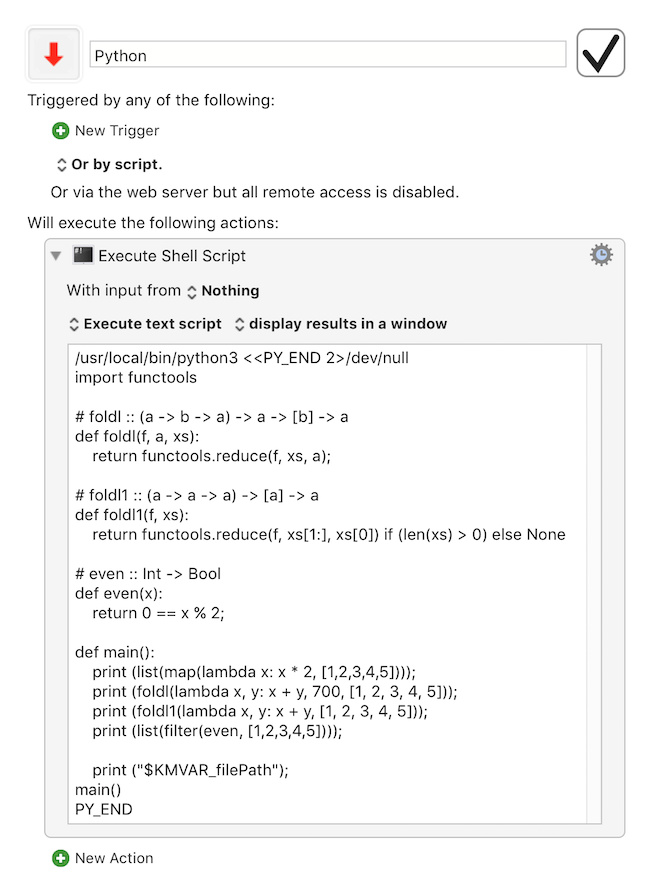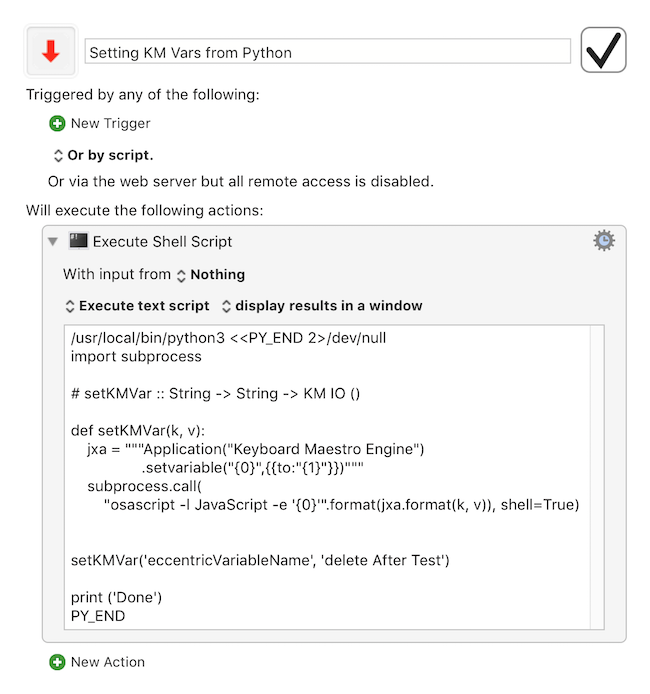# Python Example Code?

Is there a dedicated page somewhere showing how to implement a python script? I’ve seen a lot of threads, attempting to get it working. It would be great to have a best-practices example or two.

• I want to pass vars to and from KM to a python script.
• I want to call different functions in python.

Python- hello world.kmmacros (20 KB)

Cheers,
-alain

4 Likes

A post was split to a new topic: Creating SymLink to Python3 Library

As a very late follow-up to that example, here is one approach to reading KM variables (see the reference to a KM variable named filePath at the end.``````/usr/local/bin/python3 <<PY_END 2>/dev/null
import functools

# foldl :: (a -> b -> a) -> a -> [b] -> a
def foldl(f, a, xs):
return functools.reduce(f, xs, a);

# foldl1 :: (a -> a -> a) -> [a] -> a
def foldl1(f, xs):
return functools.reduce(f, xs[1:], xs) if (len(xs) > 0) else None

# even :: Int -> Bool
def even(x):
return 0 == x % 2;

def main():
print (list(map(lambda x: x * 2, [1,2,3,4,5])));
print (foldl(lambda x, y: x + y, 700, [1, 2, 3, 4, 5]));
print (foldl1(lambda x, y: x + y, [1, 2, 3, 4, 5]));
print (list(filter(even, [1,2,3,4,5])));

print ("\$KMVAR_filePath");
main()
PY_END
``````
1 Like

And one way of setting Keyboard Maestro variables from Python might be to write a setKMVar method like:``````/usr/local/bin/python3 <<PY_END 2>/dev/null
import subprocess

# setKMVar :: String -> String -> KM IO ()

def setKMVar(k, v):
subprocess.call(
"osascript -l JavaScript -e '{0}'"
.format(
"""Application("Keyboard Maestro Engine")
.setvariable("{0}",{{to:"{1}"}})"""
.format(k, v)
), shell=True
)

setKMVar('eccentricVariableName', 'delete After Test')

print ('Done')
PY_END
``````
2 Likes

@ComplexePoint Thanks for these interesting examples to learn and think aboutA question before a more complete answer ASAP:

Why

`````` /usr/local/bin/python3 <<PY_END 2>/dev/null
``````

which deletes error messages, of general interest to the developer, instead of

`````` /usr/local/bin/python3 <<PY_END
``````

Good question. I suppose it depends what values we want to derive from the action, and how we are obtaining them.

Perhaps it makes sense to retain the error messages at least during development, and then have the options of removing them later ?

@ComplexPoint
I tried to run an Execute Shell Script action that passes a KM variable in these statements:

```````#!/usr/local/bin/python`
`n='\$KMVAR_fname';`
`print(n);`
``````

Python is not getting the variable value, it just uses the string "\$KMVAR_fname" and therefore gets the wrong results.
More at: How Python script is invoked determines value of \$KMVAR_ Why?
Any suggestions for what I should try?

Also, what does the PY_END construction do?
Thanks

In the snippet:

``````/usr/local/bin/python3 <<PY_END 2>/dev/null
import functools

# foldl :: (a -> b -> a) -> a -> [b] -> a
def foldl(f, a, xs):
return functools.reduce(f, xs, a);

# foldl1 :: (a -> a -> a) -> [a] -> a
def foldl1(f, xs):
return functools.reduce(f, xs[1:], xs) if (len(xs) > 0) else None

# even :: Int -> Bool
def even(x):
return 0 == x % 2;

def main():
print (list(map(lambda x: x * 2, [1,2,3,4,5])));
print (foldl(lambda x, y: x + y, 700, [1, 2, 3, 4, 5]));
print (foldl1(lambda x, y: x + y, [1, 2, 3, 4, 5]));
print (list(filter(even, [1,2,3,4,5])));

print ("\$KMVAR_filePath");
main()
PY_END
``````

The string PY_END at start and end of the shell script brackets text that is to be passed to the Python interpreter.

(see under Bash "here documents")

You can still get bash interpolations inside it by using the \$ character.

That is how the line

``````print ("\$KMVAR_filePath");
``````

is working.

1 Like

@ComplexPoint Sorry I posted the wrong example above (corrected now). The screenshot below shows that I did use '\$KMVAR_fname", but I didn't get any bash interpolation.

``````/usr/local/bin/python3 <<PY_END 2>/dev/null
n="\$KMVAR_fname"
print(n)
PY_END
``````Thanks for the info about "Here document". That explains why KM interpolates "\$KMVAR_fname" inside the PY_END construction.

Meanwhile, I was able to find Python commands that work without using the PY_END construction:

``````#!/usr/local/bin/python
import os
n=os.environ['KMVAR_fname']
print(n)``````
2 Likes